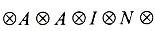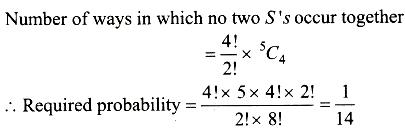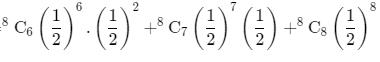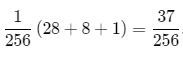Courses

# Test: Probability (CBSE Level) - 1

## 25 Questions MCQ Test Mathematics For JEE | Test: Probability (CBSE Level) - 1

Description
This mock test of Test: Probability (CBSE Level) - 1 for JEE helps you for every JEE entrance exam. This contains 25 Multiple Choice Questions for JEE Test: Probability (CBSE Level) - 1 (mcq) to study with solutions a complete question bank. The solved questions answers in this Test: Probability (CBSE Level) - 1 quiz give you a good mix of easy questions and tough questions. JEE students definitely take this Test: Probability (CBSE Level) - 1 exercise for a better result in the exam. You can find other Test: Probability (CBSE Level) - 1 extra questions, long questions & short questions for JEE on EduRev as well by searching above.
QUESTION: 1

### Probability of getting a number between 1 and 100, which is divisible by 1 and itself only, is

Solution:

Prime numbers between 1 and 100: 2, 3, 5, 7, 11, 13, 17, 19, 23, 29, 31, 37, 41, 43, 47, 53, 59, 61, 67, 71, 73, 79, 83, 89, 97 n (Prime numbers) = 25
n (Total numbers between 1 and 100) = 98
P (getting a prime number) = 25/98

QUESTION: 2

### Two players toss four coins each. The probability that both obtain the same number of heads is

Solution:

The tossing of coin by each of the two players is independent. So we can multiply the respective probabilities and get the required probability.
There are five cases:
Zero Heads
The probability that A(Let us call the players A&B) gets no head is 4C0 × (½)4 = 1/16
The probability that both of them get zero heads is 1/16×1/16 = 1/256

One Head
The probability that A gets 1 head is 4C1×1/2×(½)3 = 4×1/16 = 1/4
The probability that both A & B both get 1 head=1/4×1/4
= 1/16

Two Heads
Calculating in a similar manner,the proability that A gets two heads is,
4C2 × (½)2 × (½)2
= 3/8
The probability that both get two heads = 9/64

Three Heads
The probability that A gets 3 heads is,
4C3× (1/2)3 ×1/2
= 1/4
The probability that both get three heads = 1/16

Four Heads
The probability that A gets 4 heads is, 4C4×(½)4
= 1/16
The probability that both get four heads=1/256
So,required probability = 1/256 + 1/16 + 9/64 + 1/16 + 1/256
= 70/256
= 35/128

QUESTION: 3

### If three dice are thrown, then the probability that they show the numbers in A.P. is

Solution:

A.P. with common diff one are =4=(123)(234)(345)(456)
A.P. with common diff 2 are =2=(135)(246)
A.P. with common diff 0 are =6
⇒P=2+4+6/216=1/18

QUESTION: 4

A cubical dice has 3 on three faces, 2 on two faces and 1 on the 6 thth face .It is tossed twice. The chance that both the tosses show an even number is

Solution:

The correct option is B.

Since we have only even number 2,so when the dice is rolled twice we have (2,2) four times which means 2 on first dice pairs with both the twos on the second dice , similarly second two on first dice pairs with both the twos on the second dice

Hence the probability = 4/36 = 1/9

QUESTION: 5

A coin is tossed once. If a head comes up, then it is tossed again and if a tail comes up, a dice is thrown. The number of points in the sample space of experiment is

Solution:
QUESTION: 6

A drawer contains 5 black socks and 4 blue socks well mixed. A person searches the drawer and pulls out 2 socks at random. The probability that they match is

Solution:

Out of 9 socks, 2 can be drawn in 9C2​ ways.

Therefore, the total number of cases is 9C2​.

Two socks drawn from the drawer will match if either both are black or both are blue.

Therefore, favorable number of cases is 5C2​+ 4C2​.

Hence, the required probability is

(5C2​+ 4C2​.)/ 9C2

= 4/9

QUESTION: 7

A bag contains 5 white, 7 red and 4 black balls. Four balls are drawn one by one with replacement. The chance that atleast two balls are black is

Solution:
QUESTION: 8

A and B take turn in throwing a pair of dice. A wins if he throws a total of 5 before B throws a total of 7. If A has the first throw, the probability of his winning the game is

Solution:
QUESTION: 9

A determinant is chosen at random from the set of all determinants of order 2 with elements 0 or 1 only. The probability that the value of the determinant chosen is positive is

Solution:
QUESTION: 10

If the letters of the word ‘ INDEPENDENCE ‘ are written down at random in a row, then the chance that no two E’s occur together is

Solution:
QUESTION: 11

The letters of the word ‘ASSASSIN ‘ are written at random in a row. The chance that all the similar letters occur together is

Solution:

Total number of ways to arrange "ASSASSIN" is 8!/(4!.2!) first we fix the position.QUESTION: 12

A person throws successively with a pair of dice. The chance that he throws 9 before he throws 7 is

Solution:
QUESTION: 13

Let A be set of 4 elements. From the set of all functions from A to A, a function is chosen at random. The chance that the selected function is an onto function is

Solution:
QUESTION: 14

Both A and B throw a dice. The chance that B throws a number higher than that thrown by A is

Solution:
QUESTION: 15

Both A and B throw a dice. The chance that B throws a number not less than that thrown by A is

Solution:
QUESTION: 16

Two dice are thrown. The number of sample points in the sample space when 6 does not appear on either dice is

Solution:
QUESTION: 17

8 coins are tossed at a time. The probability of getting 6 heads up is

Solution:

he required probability  =QUESTION: 18

A dice is rolled 6 times. The probability of obtaining 2 and 4 exactly three times each is

Solution:
QUESTION: 19

A dice has 3 faces each bearing ‘ 2 ‘ and three faces each bearing ‘ 6 ‘. It is rolled once. The probability of showing up ‘a six ‘ is

Solution:

S = {2, 2, 2, 6, 6, 6}
n(S) = 6
n(6) = 3
P(showing up a 6) = n(6)/n(S) = 3/6 = 1/2

QUESTION: 20

The total area under the standard normal curve is

Solution:
QUESTION: 21

Neelam and Nidhi throw with a dice. The chance that both Neelam and Nidhi throw the same number is

Solution:
QUESTION: 22

A dice is tossed once and even number has come up. The chance that it is either 2 or 4 is

Solution:
QUESTION: 23

What is the probability of getting a sum of eight if two dice are thrown at once?

Solution:

Explanation : (1,1) (1,2) (1,3) (1,4) (1,5) (1,6) (2,1) (2,2) (2,3) (2,4)

so on upto (6,6) are the total numbers we get when we throw a pair of dice that is, 36

In the question they are asking the probability of getting a sum of 8

(2,6) (3,5) (4,4) (5,3) (6,2) are the pairs which upon adding we get 8

Therefore, 5/36 is your answer

QUESTION: 24

The probability of having atleast one head in 5 throws of a coin is

Solution:
QUESTION: 25

A fair coin is tossed a fixed number of times. If the probability of getting 4 heads is equal to the probability of getting 7 heads, then the probability of getting 2 heads is

Solution: